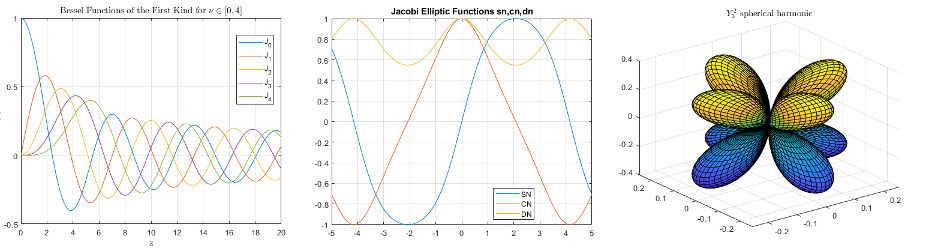Special Functions

Bessel, Legendre, elliptic, error, gamma, and other functions

Special functions are a group of well-known mathematical functions that frequently arise in real-world applications. You can use them to calculate Bessel functions, beta functions, gamma functions, error functions, elliptic integrals, and more. Since the properties of these functions have been studied extensively, you can find more information about many of them in the NIST Digital Library of Mathematical Functions.Functions

expand all

 airy Airy Functions besselh Bessel function of third kind (Hankel function) besseli Modified Bessel function of first kind besselj Bessel function of first kind besselk Modified Bessel function of second kind bessely Bessel function of second kind
 beta Beta function betainc Incomplete beta function betaincinv Beta inverse cumulative distribution function betaln Logarithm of beta function
 erf Error function erfc Complementary error function erfcinv Inverse complementary error function erfcx Scaled complementary error function erfinv Inverse error function
 gamma Gamma function gammainc Incomplete gamma function gammaincinv Inverse incomplete gamma function gammaln Logarithm of gamma function psi Digamma and polygamma functions
 ellipj Jacobi elliptic functions ellipke Complete elliptic integrals of first and second kind expint Exponential integral function legendre Associated Legendre functions